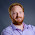## Monday, 2 July 2012

### Assignment operators

This is sort of an undocumented feature, certainly not one that I knew about until today, but as I discovered it in the documentation, I couldn't really label it as undocumented.  It's used in an example in the for loop section (which I'm investigating for a future blog post!) but not in the operators section, where I would have expected it.

In javascript there are three ways of incrementing a numeric value...

• c = c + 1
• c += 1
• c++

The first is probably the most obvious at first glance, but the other two make sense once you know what they mean.  Both + and ++ are referred to as "arithmetic operators" whereas += is referred to as an "assignment operator".

Whilst I've known this in javascript for many years, I had no idea that you could also use the following assignment operators in Uniface as well...

• Addition: c += 1 (which is the same as c = c + 1)
• Subtraction: c -= 1 (which is the same as c = c - 1)
• Multiplication: c *= 1 (which is the same as c = c * 1)
• Division: c /= 1 (which is the same as c = c / 1)
• Modulus: c %= 1 (which is the same as c = c % 1)

Interestingly in javascript if you attempt to do += with string values then it concatenates the two strings, but Uniface will always treat this as a numeric operator and attempt to cast the strings as numerics.

I've checked the performance of these shorthand operators with their longhand equivalents and they seem to perform identically, which possibly indicates that the compiler interprets them in the same way.  This means it is really a personal choice over which style the developer prefers.

It's worth noting that the arithmetic operators ++ and -- do not work - these cause compile errors.

Summary: There are shorthand numeric assignment operators available in Uniface, if you prefer this style of syntax.

#### 1 comment:

1.Uniface refers to these as "compound operators", I discovered today!